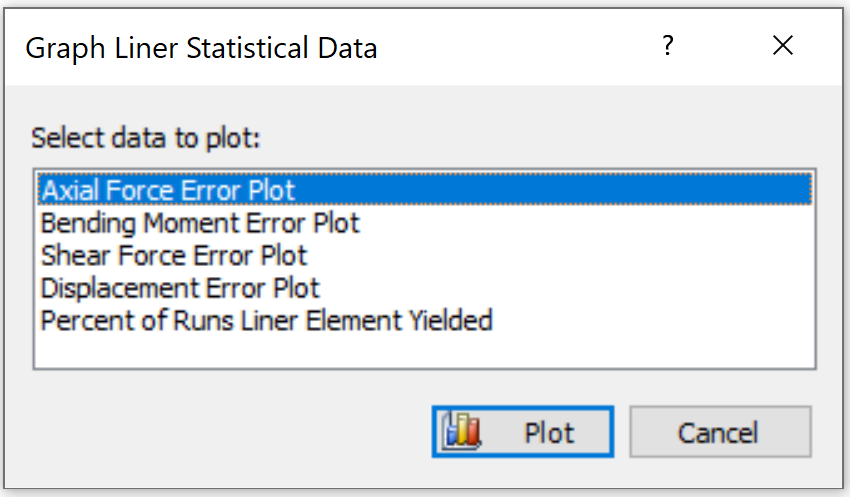# Graph Statistical Liner Data

In RS2 Interpret, the Graph Liner Statistical Data option allow you to plot statistical liner data after a probabilistic analysis. This option is only available if you have computed a Probabilistic Analysis with liners.

Five types of statistical liner data plots are available in RS2:

1. Axial Force Error Plot
2. Bending Moment Error Plot
3. Shear Force Error Plot
4. Displacement Error Plot
5. Percent of Runs Liner Element Yielded

To generate a statistical liner data plot:

1. To enable this option, make sure the files has been computed with a probabilistic analysis, and liners are modeled.
2. Select Graph Statistical Liner Data from the Statistics menu. A dialog will be prompted as below.
3.4. In the Graph Liner Statistical Data dialog, select a data type to plot.
5. Select Plot when complete selection. The graph will be generated and displayed in a new window.